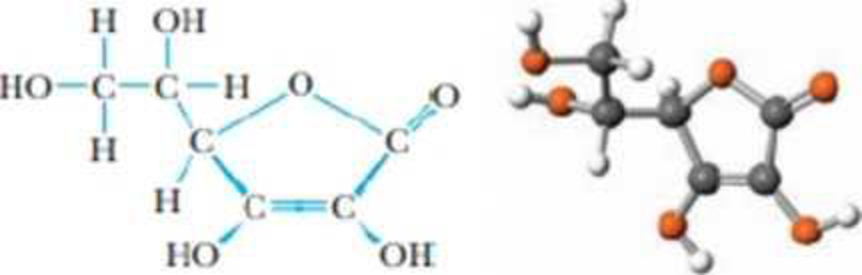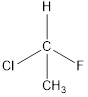Chapter 23, Problem 66PS

Chapter
Section
Textbook Problem

The structure of vitamin C, whose chemical name is ascorbic acid, is drawn below (without lone pairs of electrons).(a) What is the approximate value for the O—C—O bond angle?(b) There are four OH groups in this structure. Estimate the C—O—H bond angles for these groups. Will they be the same value (more or less), or should there be significant differences in these bond angles?(c) Is the molecule chiral? How many chiral carbon atoms can be identified in this structure?(d) Identify the shortest bond in this molecule.(e) What are the functional groups of the molecule?

(a)

Interpretation Introduction

Interpretation:

The approximate value for the O - C - O bond angle has to be determined.

Concept introduction:

Hybridization is the mixing of valence atomic orbitals to get equivalent hybridized orbitals that having similar characteristics and energy.

Geometry of a molecule can be predicted by knowing its hybridization.

sp3  hybrid orbitals is produced by hybridization of single s-orbital and three p-orbital. It has tetrahedral geometry

sp2  hybrid orbitals is produced by hybridization between one s-orbital and two p-orbitals. It has trigonal-planar geometry

sp  hybrid orbitals is produced by hybridization of single s-orbital and single p-orbital. It has linear geometry

Explanation

The structure of vitamin C is,

In OCO, the carbon atom is sp

(b)

Interpretation Introduction

Interpretation:

The bond angles for the four -OH groups have to be estimated.

Concept introduction:

Hybridization is the mixing of valence atomic orbitals to get equivalent hybridized orbitals that having similar characteristics and energy.

Geometry of a molecule can be predicted by knowing its hybridization.

sp3  hybrid orbitals is produced by hybridization of single s-orbital and three p-orbital. It has tetrahedral geometry

sp2  hybrid orbitals is produced by hybridization between one s-orbital and two p-orbitals. It has trigonal-planar geometry

sp  hybrid orbitals is produced by hybridization of single s-orbital and single p-orbital. It has linear geometry

(c)

Interpretation Introduction

Interpretation:

The chiral carbon in the molecule has to be determined

Concept introduction:

A chiral carbon is the carbon atom which is substituted to four different groups. Due to presence of chiral carbon a molecule become chiral and it gives optical isomers also that is R and S isomers. For example,All the substituents are different hence is is a chiral carbon and so molecule.

(d)

Interpretation Introduction

Interpretation:

The shortest bond in the molecule has to be determined

Concept introduction:

Bond order: It is the measure of number of electron pairs shared between two atoms.

Bondorder=12(NumberofelectronsinbondoingMOs-NumberofelectronsinantibondingMOs)

Bond length is inversely proportional to the bond order.

A higher bond order results in a shorter bond for a given pair of atoms.

(e)

Interpretation Introduction

Interpretation:

The functional group in the molecule has to be identified.

Concept introduction:

A chiral carbon is the carbon atom which is substituted to four different groups. Due to presence of chiral carbon a molecule become chiral and it gives optical isomers also that is R and S isomers. For example,All the substituents are different hence is is a chiral carbon and so molecule.

Still sussing out bartleby?

Check out a sample textbook solution.

See a sample solution

The Solution to Your Study Problems

Bartleby provides explanations to thousands of textbook problems written by our experts, many with advanced degrees!

Get Started

Name the following compounds. a Sn3(PO4)2 b NH4NO2 c Mg(OH)2 d NiSO3

General Chemistry - Standalone book (MindTap Course List)

Name one environmental factor that typically influences enzyme function.

Biology: The Unity and Diversity of Life (MindTap Course List)

Most water-soluble vitamins are not stored in tissues to any great extent. T F

Nutrition: Concepts and Controversies - Standalone book (MindTap Course List)

Where would you look for water in our solar system?

Oceanography: An Invitation To Marine Science, Loose-leaf Versin